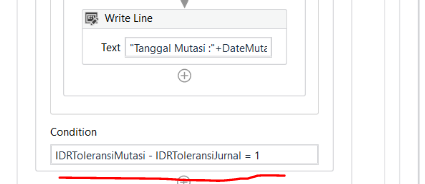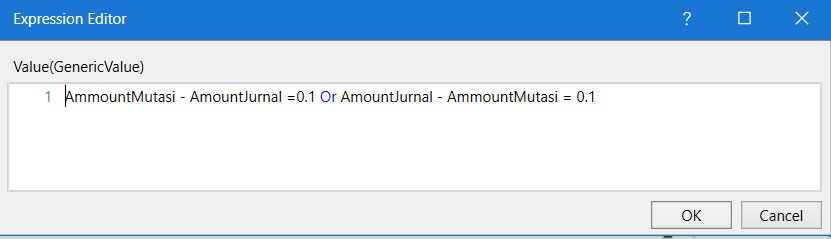# Substract String with value Currecny

I have a problem when I want to reduce the currency value but the value is assigned in the form of a string, how can I reduce the value of the currency in the form of a string?

whats the value of `IDRToleransiMutasi`?

im assign with value = MappingRow2(“Transaction Amount”)

can you change thisto this
`cdbl(IDRToleransiMutasi) - cdbl(IDRToleransiMutasi) = 1`

okay I’ll try, thanks for the help, but I still don’t understand what the “cdbl” function is used for

and data type IDRToleransiMutasi generic value or string??

converts string to number so that you can subtract it

whats the current type? you can run the program first and see if it worksim use generic value type. btw im use this assign and failed, why?

if you do subtraction then replace AmmountMutasi with `cdbl(AmmountMutasi)`

`cdbl(AmmountMutasi) - cdbl(AmmountMutasi) = 0.1 or cdbl(AmountJurnal) - cdbl(AmountJurnal) = 0.1`